## Simple plots

As it was already mentioned, mdatools has its own functions for plotting with several extra options not available in basic plot tools. These functions are used to make all plots in the models and results (e.g. scores, loadings, predictions, etc.) therefore it can be useful to spend some time and learn the new features (e.g. coloring data points with a vector of values or using manual ticks for axes). But if you are going to make all plots manually (e.g. using ggplot2) you can skip this and the next sections.

In this section we will look at how to make simple plots from your data objects. Simple plots are scatter (type = "p"), line (type = "l"), line-scatter (type = "b"), bar (type = "h") or errorbar (type = "e") plots made for a one set of objects. All plots can be created using the same method mdaplot() by providing a whole dataset as a main argument. Depending on a plot type, the method “treats” the data values differently.

This table below contains a list of parameters for mdaplot(), which are not available for traditional R plots. In this section we will describe most of the details using simple examples.

Parameter Description
cgroup a vector of values (same as number of rows in data) used to colorize plot objects with a color gradient.
colmap color map for the color gradient (possible values are 'default', 'gray' or a vector with colors).
show.colorbar when color grouping is used, mdaplot() shows a color bar legend, this parameter allows to turn it off.
show.labels logical parameter showing labels beside plot objects (points, lines, etc). Size and color of labels can be adjusted using parameters lab.cex and lab.col.
labels parameter telling what to use as labels (by default row names, but can also be indices or manual values).
lab.col color for the labels.
lab.cex font size for the labels (as a scale factor).
xticks vector with numeric values to show the x-axis ticks at.
yticks vector with numeric values to show the y-axis ticks at.
xticklabels vector with labels (numbers or text) for the x-ticks.
yticklabels vector with labels (numbers or text) for the y-ticks.
xlas an integer between 0 and 3 telling at which angle the x-tick labels have to be shown.
ylas an integer between 0 and 3 telling at which angle the y-tick labels have to be shown.
show.axes logical, if TRUE, function will make a new plot, if FALSE, add the plot objects to a previous one.
show.lines a vector with two numbers — position of horizontal and vertical lines on a plot (e.g. coordinate axes).
show.grid logical, show or not a grid. It places grid behind the plot object in contrast to conventional grid() method. Use grid.lwd and grid.col parameters to adjust the grid look.
show.excluded logical, show or not points or lines corresponded to the excluded rows.
opacity opacity of colors in range 0…1 (applied to all colors of current plot).

### Scatter plots

We will use people dataset for illustration how scatter plots work (see ?people for details).

data(people)
attr(people, "name") <- "People"
attr(people, "xaxis.name") <- "Variables"
attr(people, "yaxis.name") <- "Persons"

For scatter plots the method takes first two columns of a dataset as x and y vectors. If only one column is available mdaplot() uses it for y-values and generate x-values as an index for each value.

par(mfrow = c(1, 2))
mdaplot(people, type = "p")
mdaplot(mda.subset(people, select = c(6, 7)), type = "p")All parameters, available for the standard points() method will work with mdaplot() as well. Besides that, you can colorize points according to some values using a color gradient. By default, the gradient is generated using one of the diverging color schemes from colorbrewer2.org, but this can be changed using parameter colmap as it is shown below.

par(mfrow = c(2, 2))
mdaplot(people, type = "p", cgroup = people[, "Beer"])
mdaplot(people, type = "p", cgroup = people[, "Beer"], show.colorbar = FALSE)
mdaplot(people, type = "p", cgroup = people[, "Beer"], colmap = "gray")
mdaplot(people, type = "p", cgroup = people[, "Beer"], colmap = c("red", "yellow", "green"))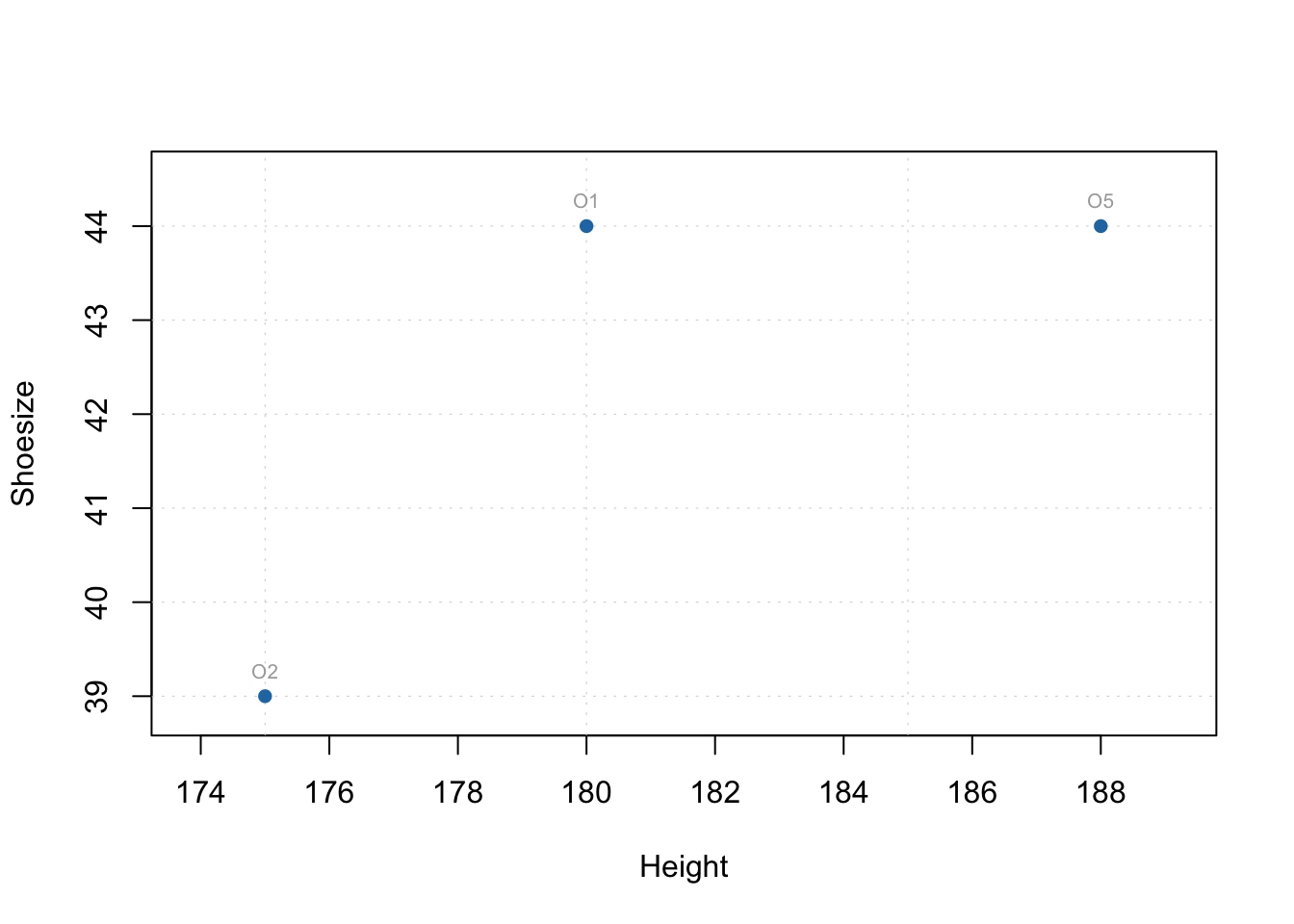If the vector with values for color grouping is a factor, level labels will be shown on a colorbar legend and there will be a small margin between bars.

g = factor(people[, "Sex"], labels = c("Male", "Female"))

par(mfrow = c(1, 2))
mdaplot(people, type = "p", cgroup = g)
mdaplot(people, type = "p", cgroup = g, colmap = "gray")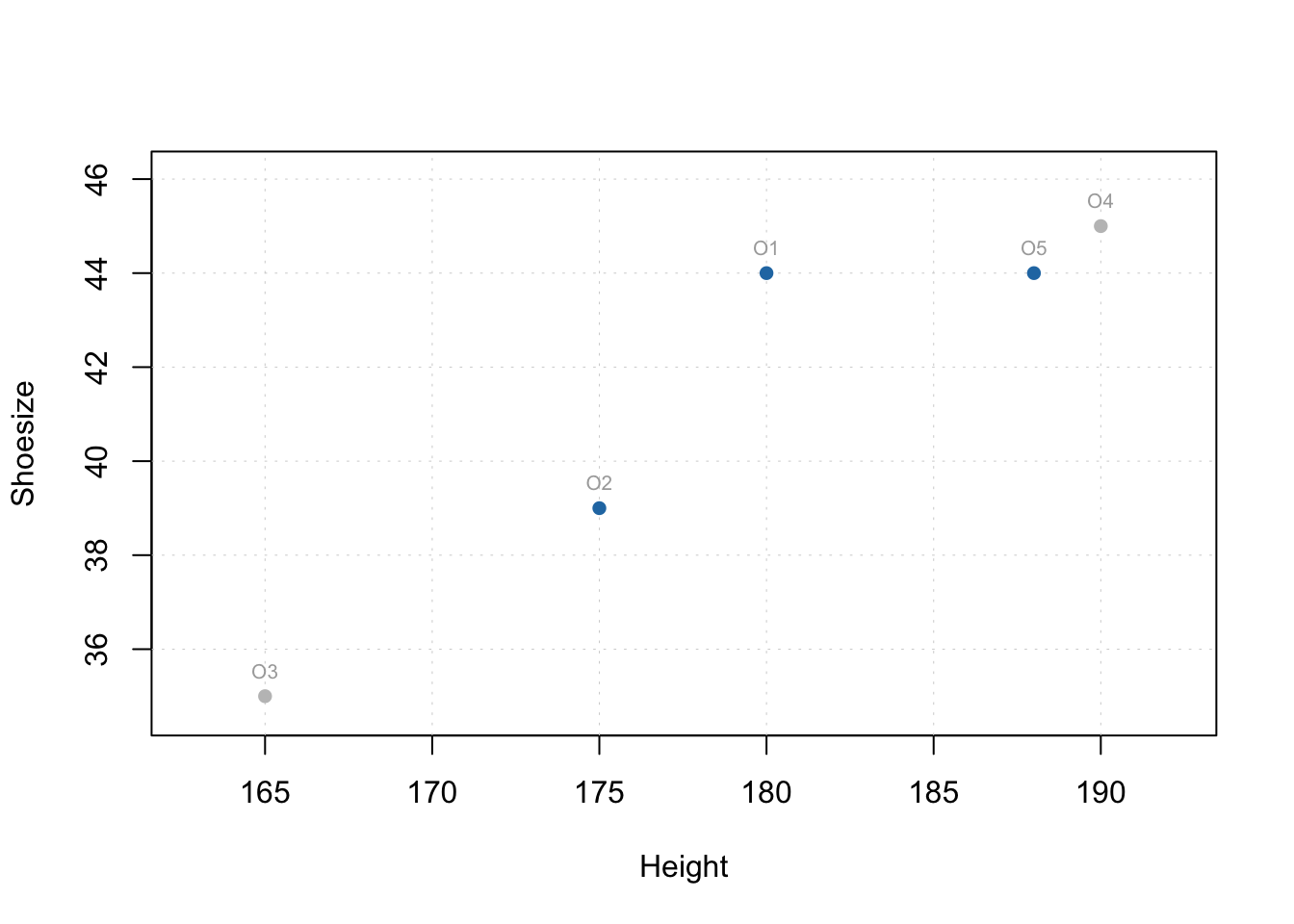If you use point characters from 21 to 25 (the ones which allow to specify both color of border and background of the marker symbol) you can use pch.colinv to apply color grouping to background instead of border. See an example below

par(mfrow = c(1, 2))
mdaplot(people, type = "p", cgroup = people[, "Beer"], pch = 21, bg = "white")
mdaplot(people, type = "p", cgroup = people[, "Beer"], pch = 21, bg = "white", pch.colinv = TRUE)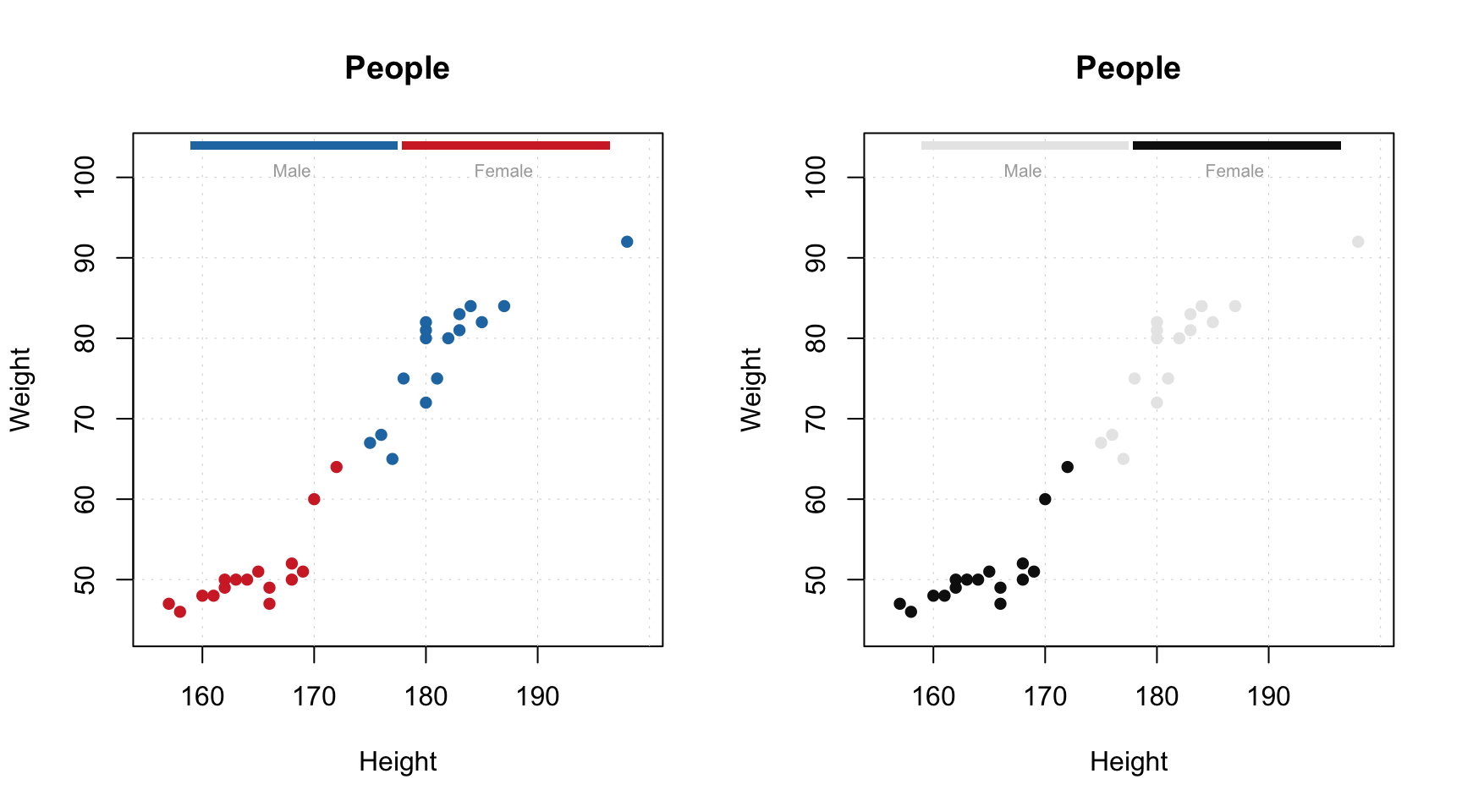Another useful option is adding labels to the data points. By default row names will be taken for the labels but you can specify a parameter labels, which can be either a text ("names" or "indices") or a vector with values to show as labels. Color and size of the labels can be adjusted.

par(mfrow = c(2, 2))
mdaplot(people, type = "p", show.labels = TRUE)
mdaplot(people, type = "p", show.labels = TRUE, labels = "indices")
mdaplot(people, type = "p", show.labels = TRUE, labels = "names", lab.col = "red", lab.cex = 0.5)
mdaplot(people, type = "p", show.labels = TRUE, labels = paste0("O", seq_len(nrow(people))))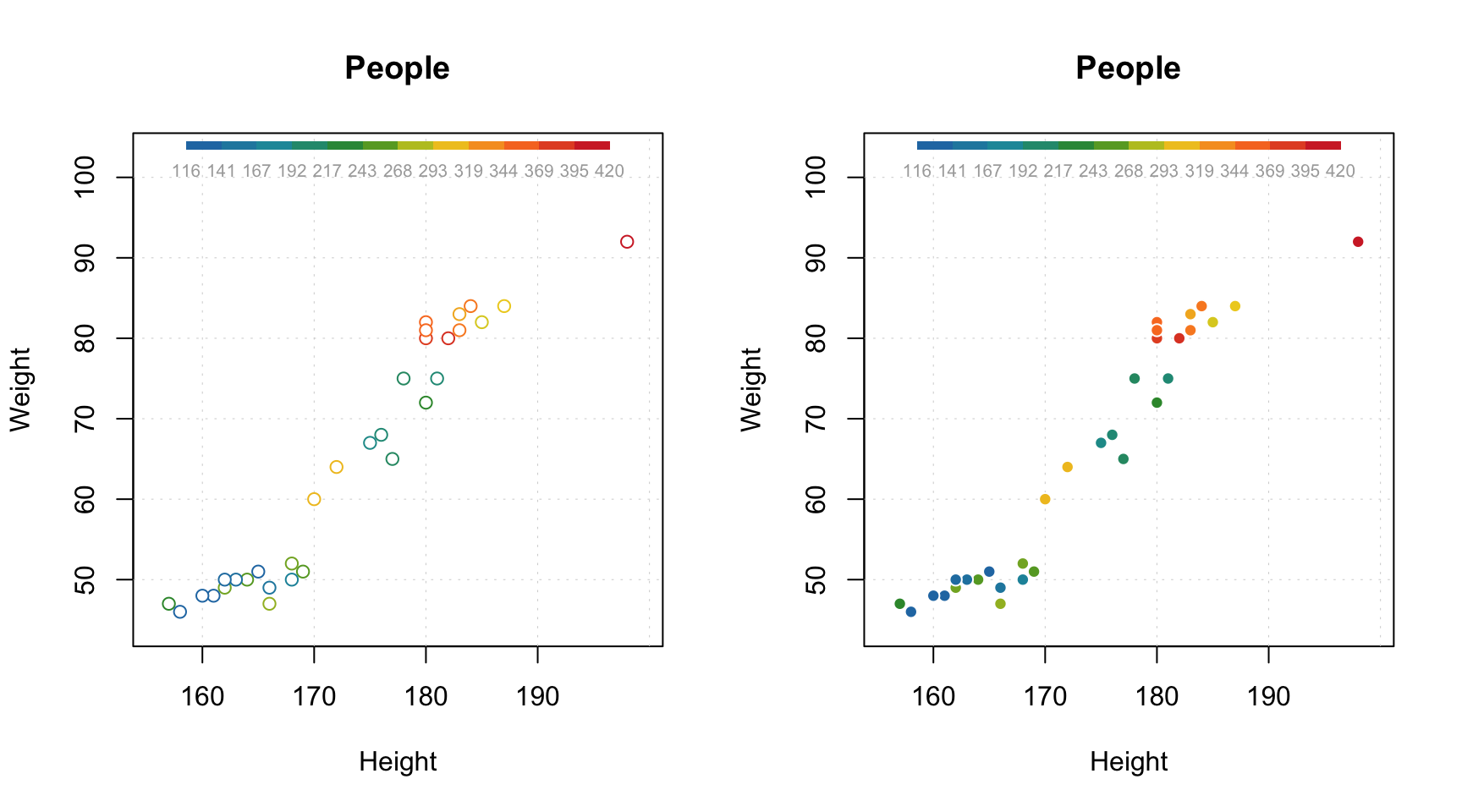To avoid any problems with arguments when you make a subset, use mda.subset() instead of the traditional ways. As you can see in the example below, if we take first 16 rows, information about excluded objects (as well as all other uder defined arguments, e.g. "name") disappear and they are show in the plot as normal. But if we use mda.subset() it will take the subset without excluded rows as it is shown below. The subset can be created using logical expressions as well as indices or names of the rows.

weight = people[, "Weight"]
par(mfrow = c(2, 2))
mdaplot(people[1:16, ], show.labels = TRUE)
mdaplot(mda.subset(people, subset = 1:16), show.labels = TRUE)
mdaplot(mda.subset(people, subset = c("Lisa", "Benito", "Federico")), show.labels = TRUE)
mdaplot(mda.subset(people, subset = weight > 70), show.labels = TRUE)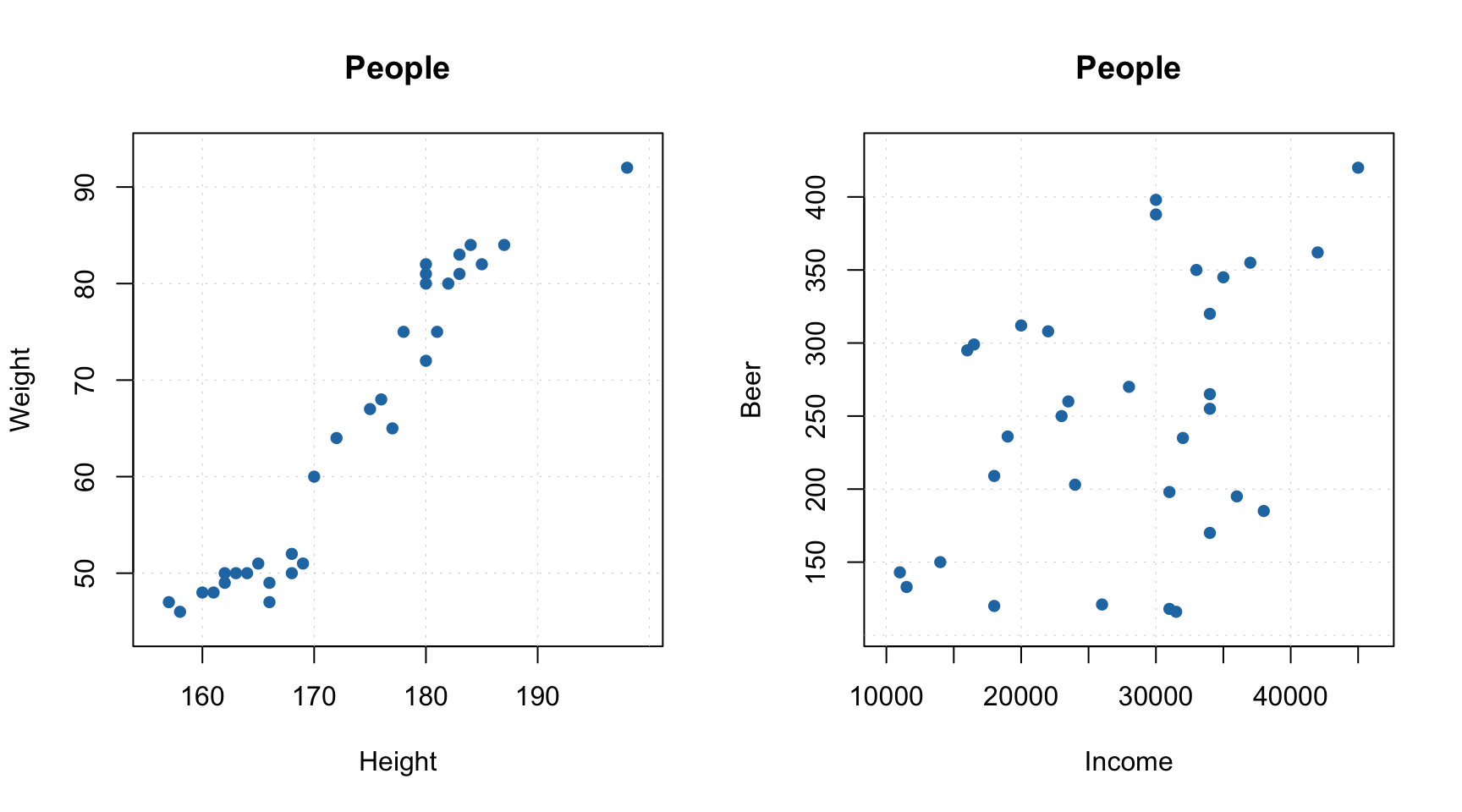You can also manually specify axis ticks and tick labels. The labels can be rotated using parameters xlas and ylas, see the examples below.

par(mfrow = c(2, 2))
mdaplot(people, xticks = c(165, 175, 185), xticklabels = c("Small", "Medium", "Hight"))
mdaplot(people, yticks = c(55, 70, 85), yticklabels = c("Light", "Medium", "Heavy"))
mdaplot(people, xticks = c(165, 175, 185), xticklabels = c("Small", "Medium", "Hight"), xlas = 2)
mdaplot(people, yticks = c(55, 70, 85), yticklabels = c("Light", "Medium", "Heavy"), ylas = 2)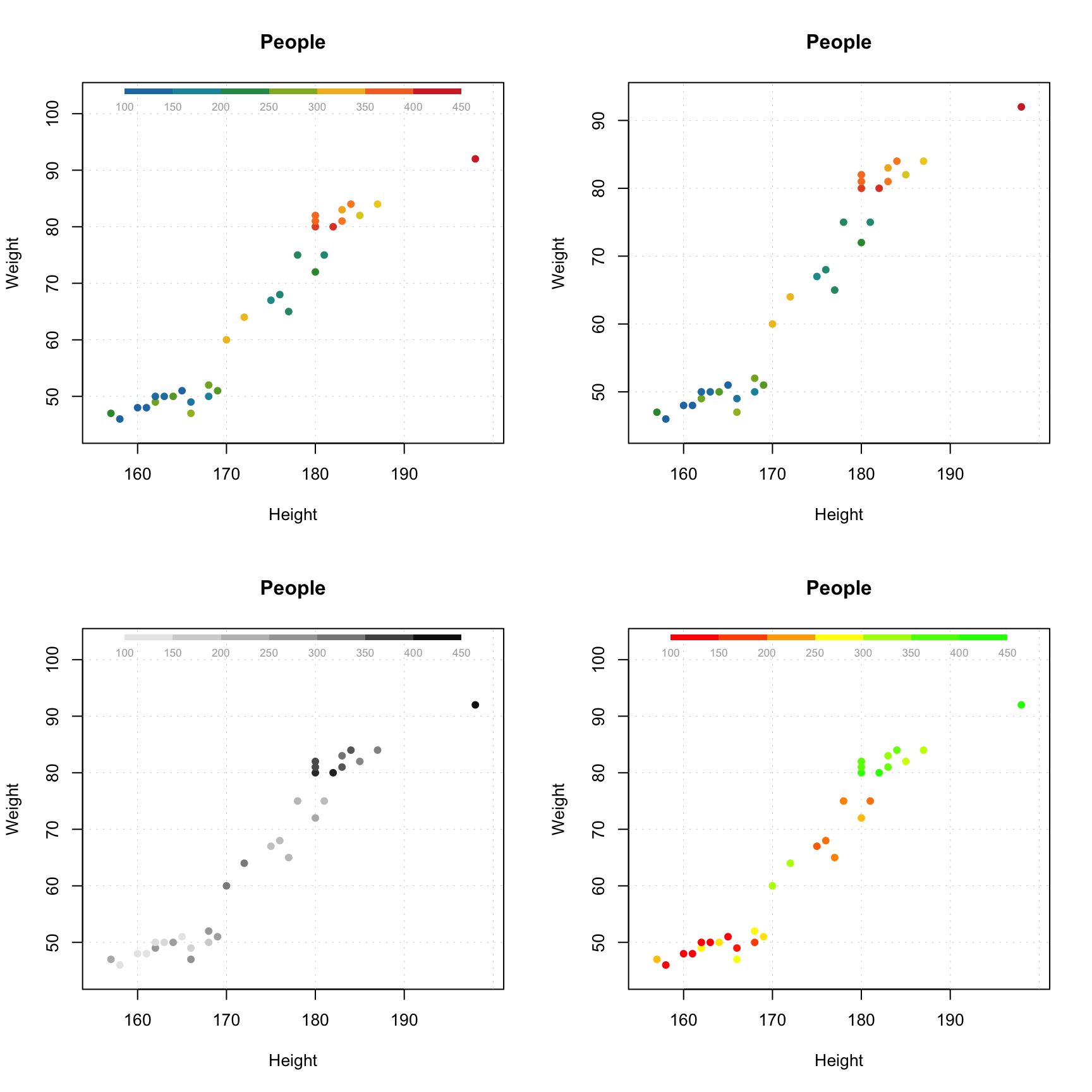If both axis labels and rotated axis ticks have to be shown, you can adjust plot margins and position of the label using par() function and mtext() for positioning axis label manually.

par(mfrow = c(1, 2))

# change margin for bottom part
par(mar = c(6, 4, 4, 2) + 0.1)
mdaplot(people, xticks = c(165, 175, 185), xticklabels = c("Small", "Medium", "Hight"),
xlas = 2, xlab = "")
mtext("Height", side = 1, line = 5)

# change margin for left part
par(mar = c(5, 6, 4, 1) + 0.1)
mdaplot(people, yticks = c(55, 70, 85), yticklabels = c("Light", "Medium", "Heavy"),
ylas = 2, ylab = "")
mtext("Weight", side = 2, line = 5)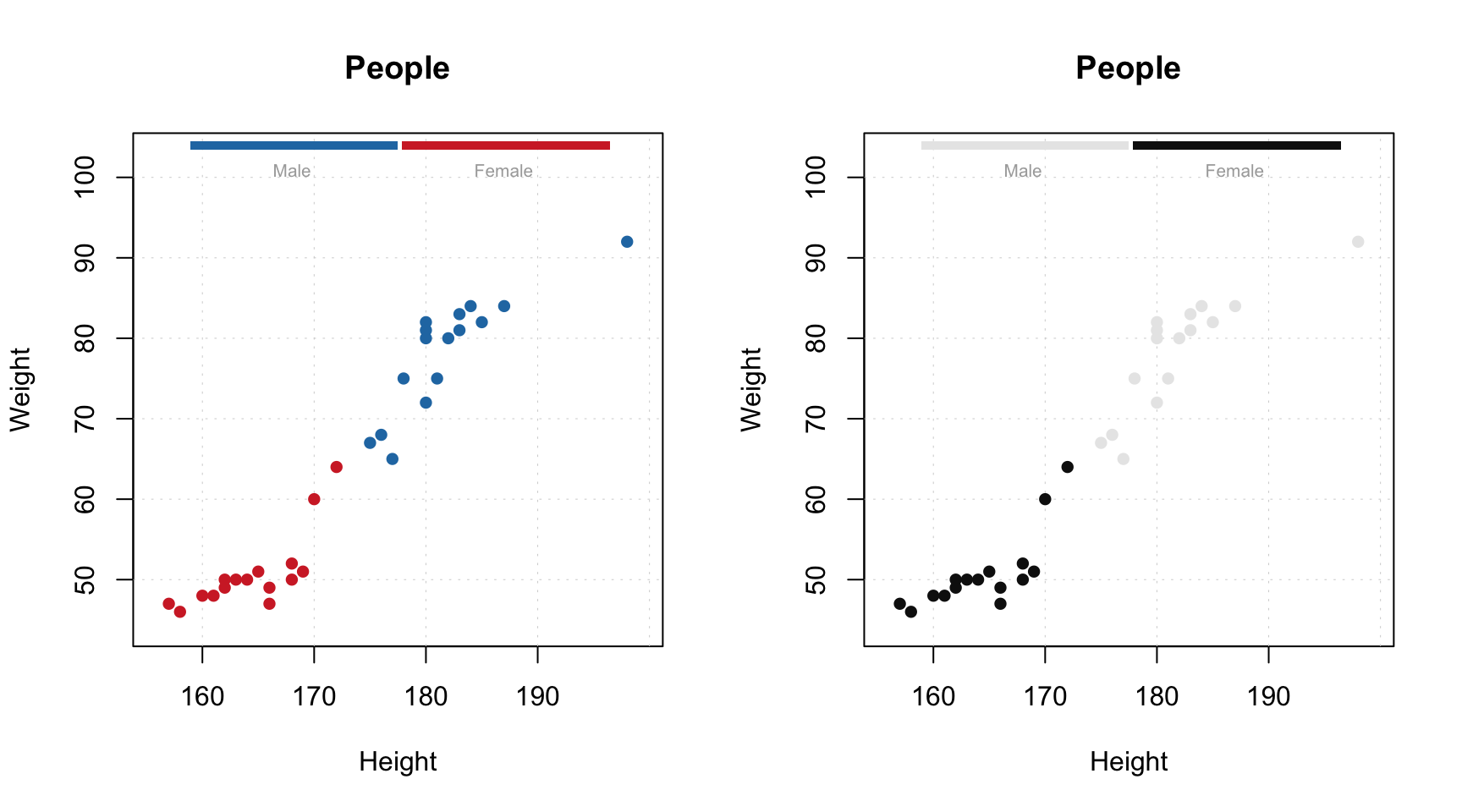There is also a couple of other parameters, allowing to show/hide grid as well as show horizontal and vertical lines on the plot (axes limits will be adjusted correspondingly).

par(mfrow = c(1, 2))
mdaplot(people, show.grid = FALSE, show.lines = c(170, 65))
mdaplot(people, show.lines = c(220, NA))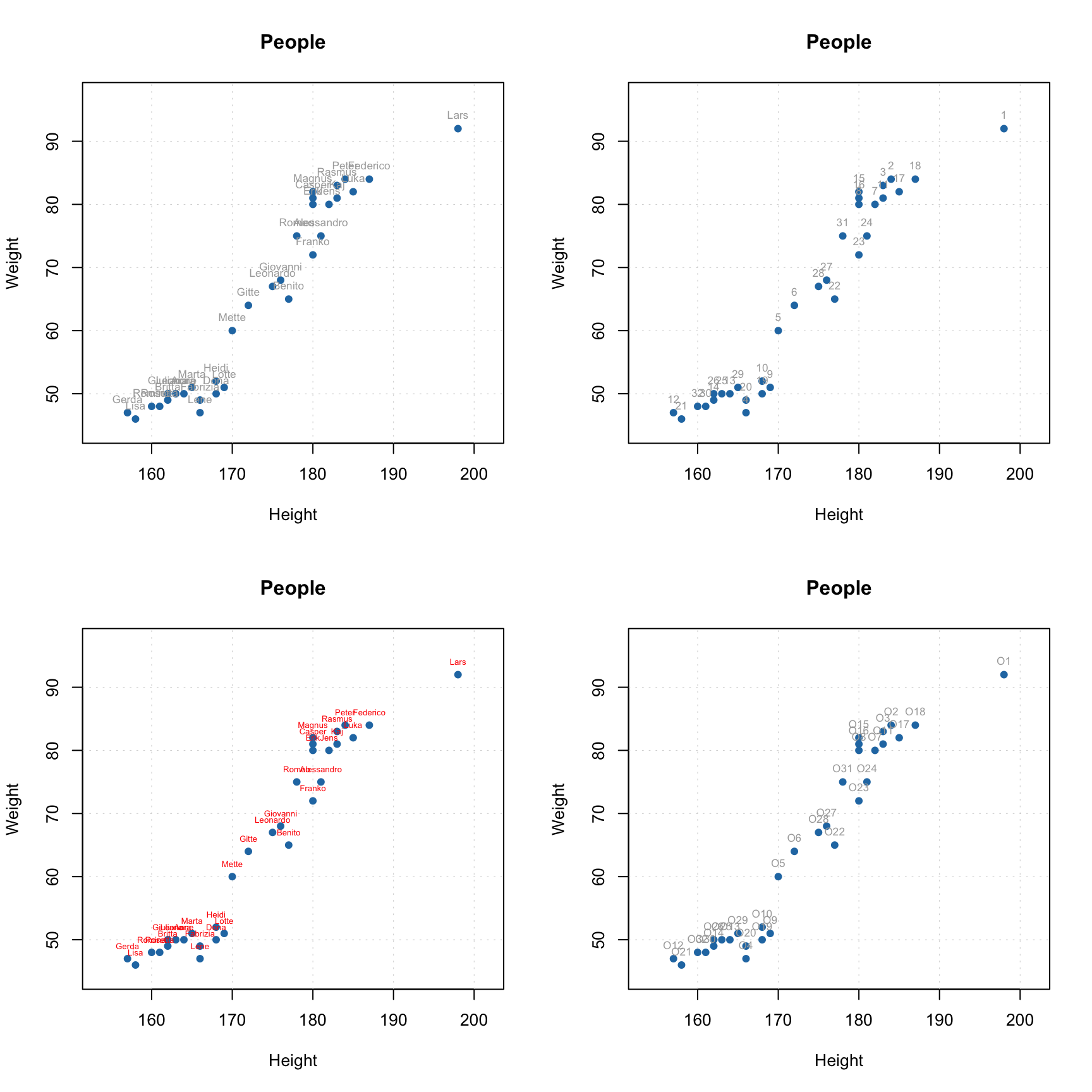From version 0.10.0 function mdaplot() returns plot series data, which can be used for extra options. For example, in case of scatter plot you can add confidence ellipse or convex hull for data points. To do this, points must be color grouped by a factor as shown below. For confidence ellipse you can specify the confidence level (default 0.95).

g = factor(people[, "Sex"], labels = c("M", "F"))

par(mfrow = c(1, 2))
p = mdaplot(people, cgroup = g)
plotConvexHull(p)
p = mdaplot(people, cgroup = g)
plotConfidenceEllipse(p, conf.level = 0.90)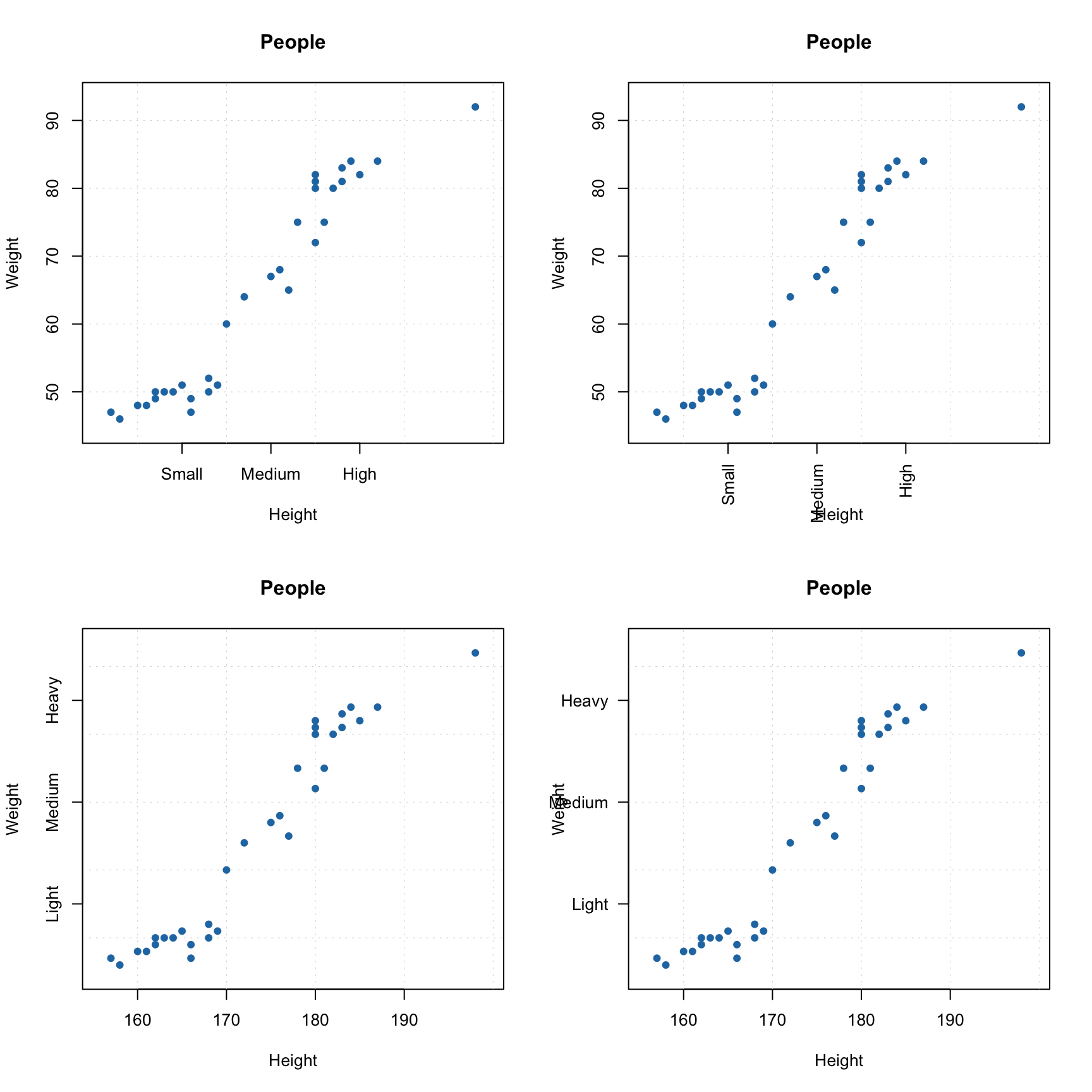In case when number of data points is large (e.g. when dealing with images, where every pixel is a data point), using density plot is a good alternative to conventional scatter plots. The plot does not show all data points but instead split the whole plot space into small hexagonal regions and use color gradient for illustration a density of the points in each region. This approach is known as hexagonal binning. To create a density plot simply use type="d". You can also specify color map and number of bins along each axes (nbins).

The code below show an example of using density plots for 100000 data points with x and y values taken from normally distributed population.

x = rnorm(100000)
y = rnorm(100000)
d = cbind(x, y)

par(mfrow = c(2, 2))
mdaplot(d, type = "d")
mdaplot(d, type = "d", nbins = 80)
mdaplot(d, type = "d", colmap = "gray")
mdaplot(d, type = "d", nbins = 80, colmap = c("blue", "red"))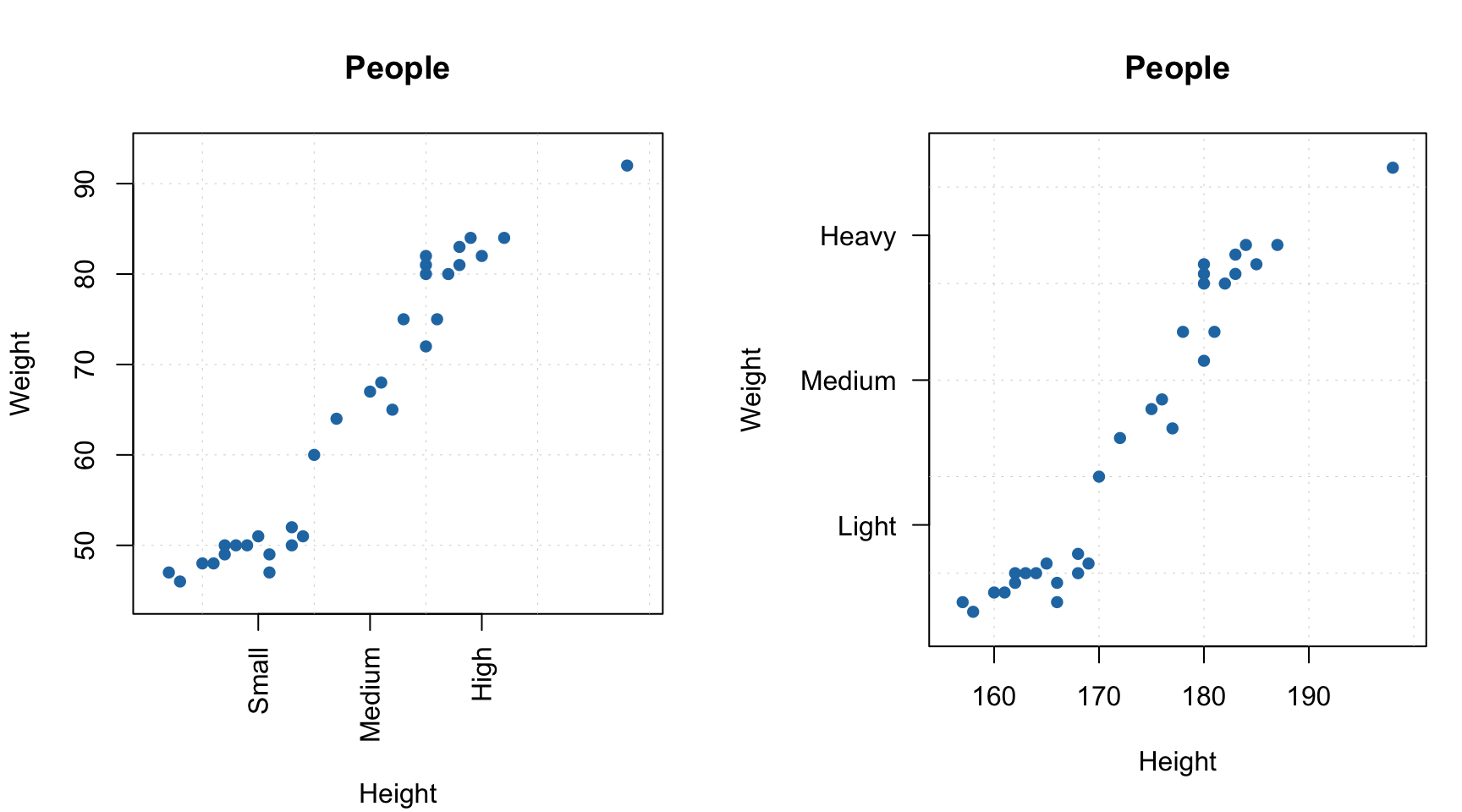### Line plots

When line plot is created, the mdatools() shows a line plot for every row. So if data set has more than one row, the plot will show a banch of lines having same properties (color, type, etc). This is particularly useful when working with signals and spectroscopic data. In this subsection we will use simulated UV/Vis spectra from simdata.

data(simdata)
spectra = simdata$spectra.c conc = simdata$conc.c[, 1]
wavelength = simdata\$wavelength

attr(spectra, "name") = "UV/Vis spectra"
attr(spectra, "xaxis.name") = "Band index"

Here are simple examples of how to make the line plots.

par(mfrow = c(2, 1))
mdaplot(spectra, type = "l")
mdaplot(spectra, type = "l", col = "darkgray", lty = 2)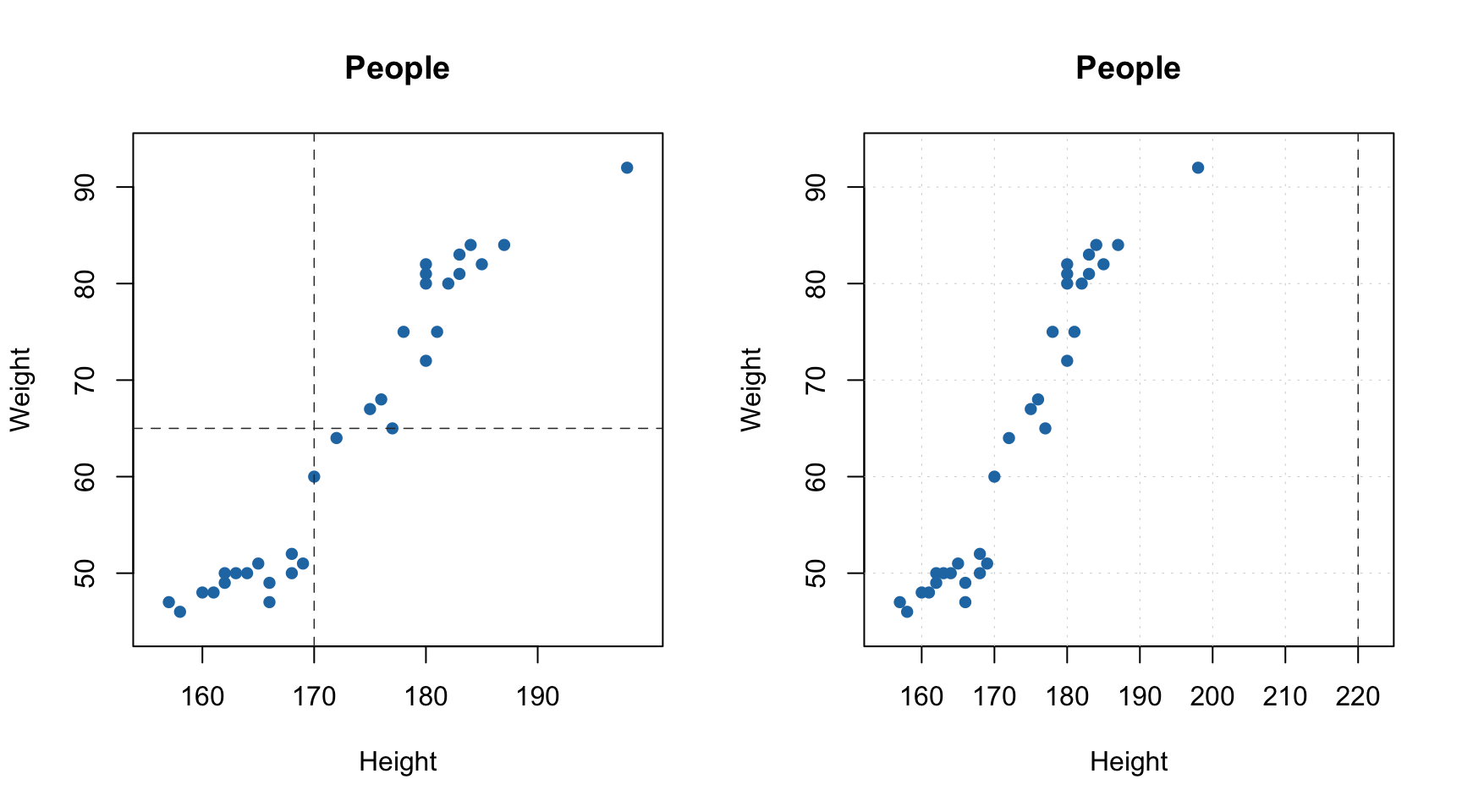Most of the parameters described for scatter plots will work for the line plots as well. For example, you can colorise the lines by using a vector with some values (in the example below I use concentration of one of the chemical components).

par(mfrow = c(1, 1))
mdaplot(spectra, type = "l", cgroup = conc)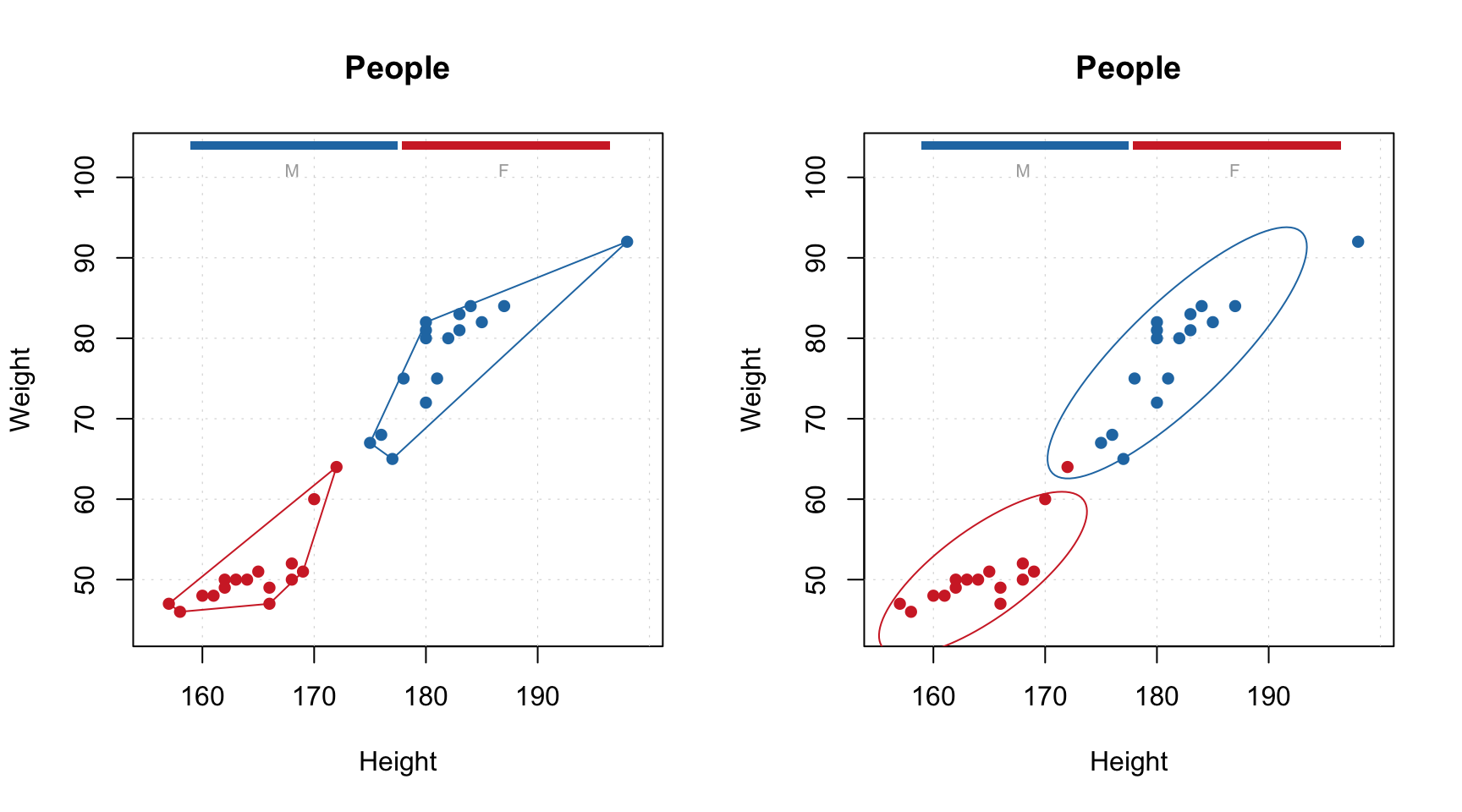One of the new features, appeared first in version 0.8.0, is a special attribute, allowing to provide manual x-values — 'xaxis.values' (similar parameter for y-values is 'yaxis.values'). In the example below we show the spectra using wavelength in nm and wavenumbers in inverse cm.

par(mfrow = c(2, 1))

attr(spectra, "xaxis.name") = expression("Wavenumbers, cm"^-1)
attr(spectra, "xaxis.values") = 10^7/wavelength
mdaplot(spectra, type = "l")

attr(spectra, "xaxis.name") = "Wavelength, nm"
attr(spectra, "xaxis.values") = wavelength
mdaplot(spectra, type = "l")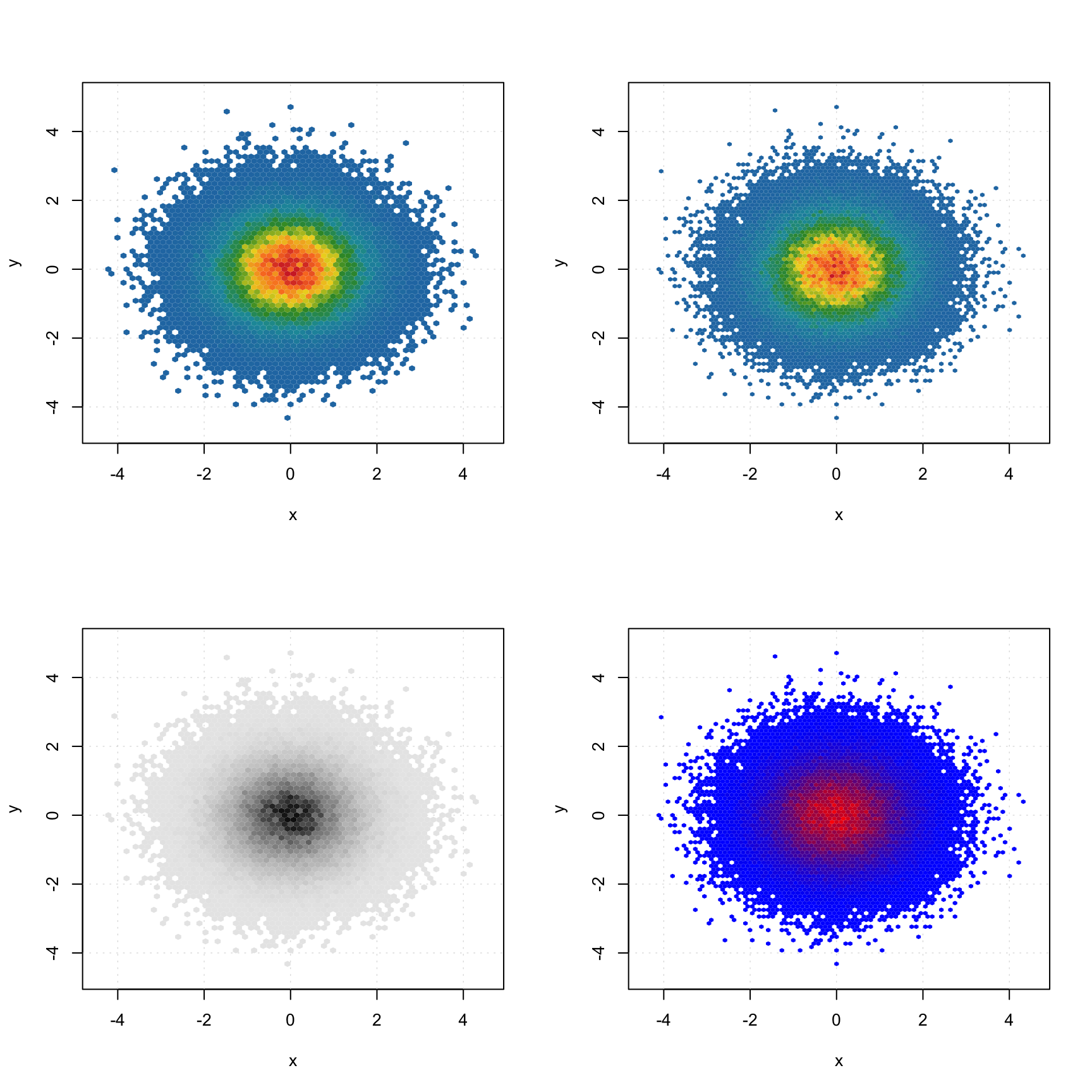When you provide such data to any model methods (e.g. PCA, PLS, etc), then all variable related results (loadings, regression coefficients, etc.) will inherit this attribute and use it for making line plots.

### Bar and errorbar plots

Bar plot is perhaps the simplest as it shows values for the first row of the data as bars. Let us get back to the people data, calculate mean for all variables and show the calculated values as a bar plot (excluding column with Income as it has much bigger values comparing to the others) — in the simplest form as well as with some extra parameters.

m = matrix(apply(people, 2, mean), nrow = 1)
colnames(m) = colnames(people)
m = mda.exclcols(m, "Income")
attr(m, "name") = "People (means)"

par(mfrow = c(2, 1))
mdaplot(m, type = "h")
mdaplot(m, type = "h", xticks = 1:12, xticklabels = colnames(people), col = "red",
show.labels = TRUE, labels = "values")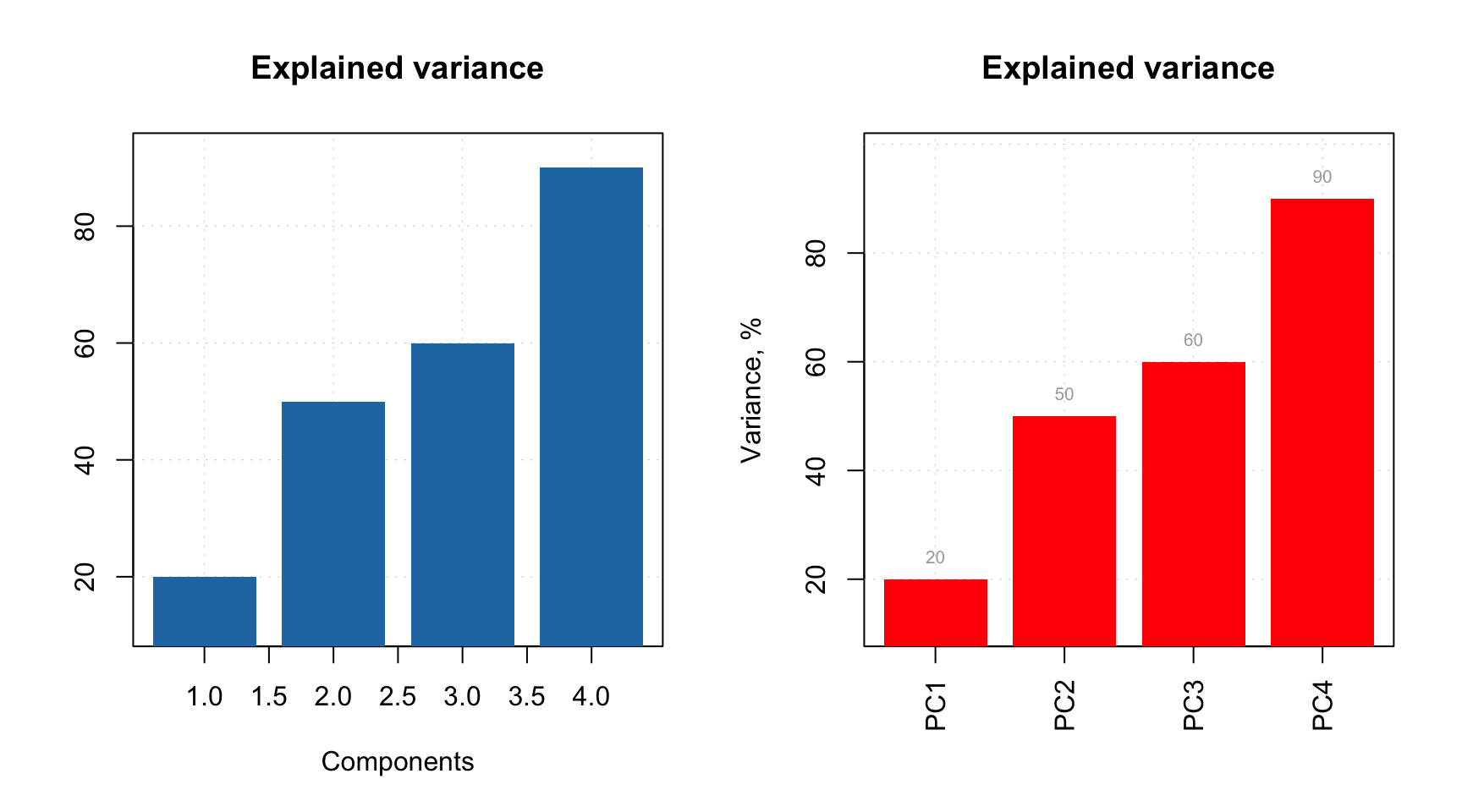Errorbar plot always expect data to have two or three rows. The first row is a origin points of the error bars, secod row is the size of the bottom part and the third row is the size of the top part. If data has only two rows the both parts will be symmetric related to the origin. In the example below we show mean and standard deviation of the people data as an error bar.

d = rbind(apply(people, 2, mean), apply(people, 2, sd))
rownames(d) = c("Mean", "Std")
colnames(d) = colnames(people)
attr(d, 'name') = "Statistics"
d = mda.exclcols(d, "Income")

par(mfrow = c(2, 1))
mdaplot(d, type = "e")
mdaplot(d, type = "e", xticks = 1:12, xticklabels = colnames(people), col = "red")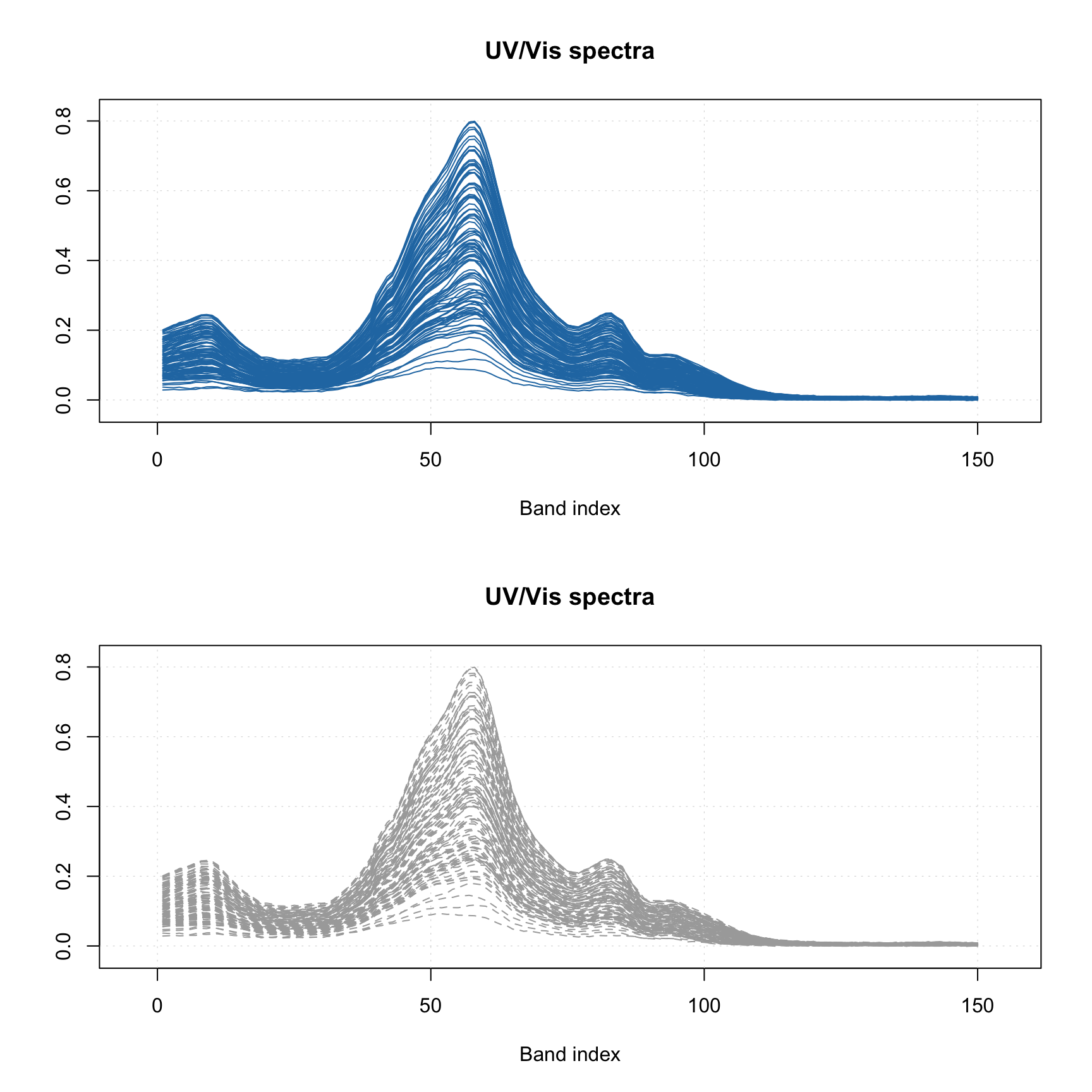All simple plots can be combined together on the same axes. In this case, first plot is created as usual and all other plots have to be created with option show.axes = FALSE as it is shown below. It must be noted that in this case axes limits have to be set manually when creating the first plot.

par(mfrow = c(2, 1))

mdaplot(m, type = "h", col = "lightgray", ylim = c(0, 400))
mdaplot(d, type = "e", show.axes = FALSE, pch = NA)

mdaplot(m, type = "b", ylim = c(0, 400))
mdaplot(d, type = "e", show.axes = FALSE)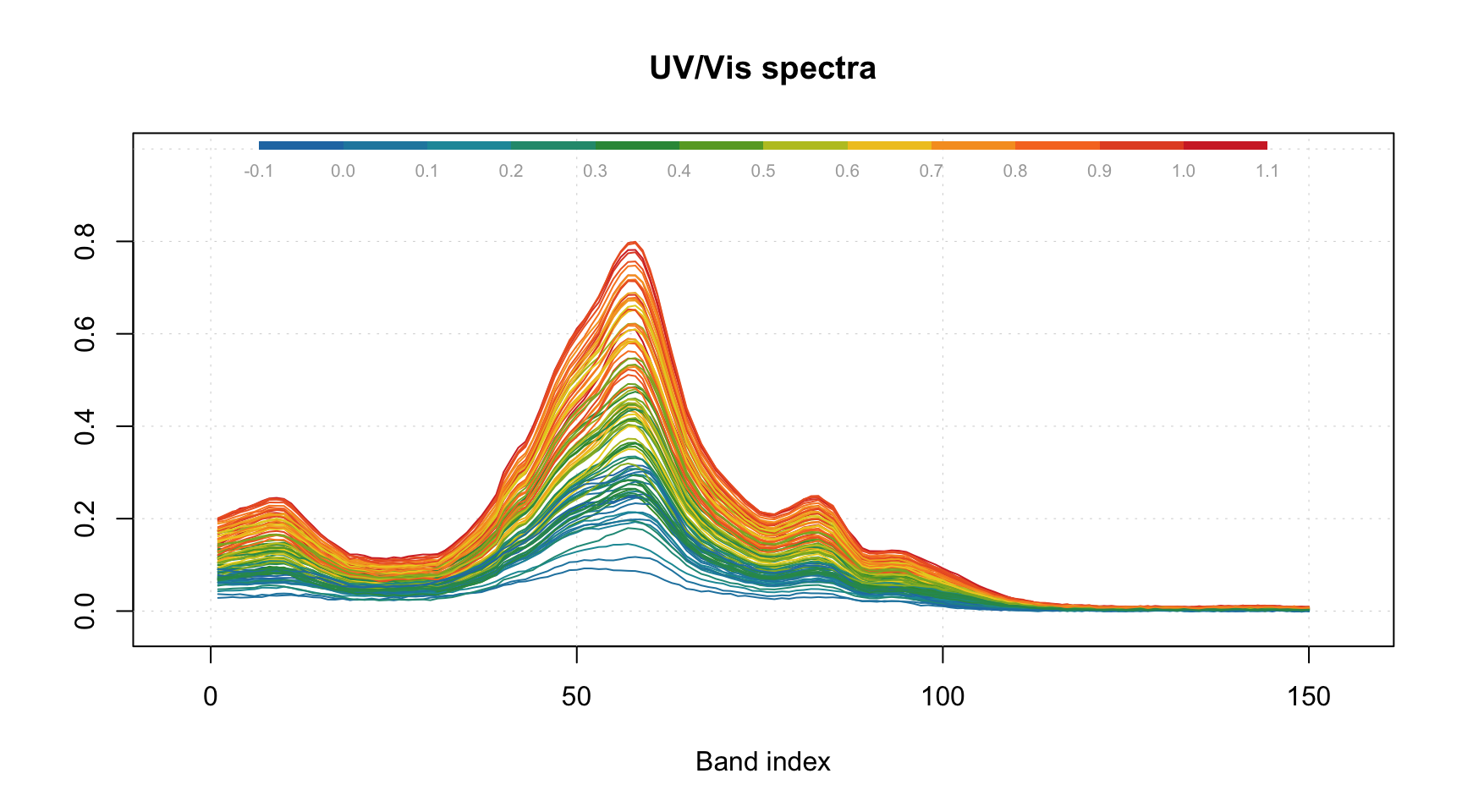In the next section we will discuss plots for several groups of objects (rows).